• # 『Python』内存分析_list和array

## 零、预备知识

在Python中，列表是一个动态的指针数组，而array模块所提供的array对象则是保存相同类型的数值的动态数组。由于array直接保存值，因此它所使用的内存比列表少。列表和array都是动态数组，因此往其中添加新元素，而没有空间保存新的元素时，它们会自动重新分配内存块，并将原来的内存中的值复制到新的内存块中。为了减少重新分配内存的次数，通常每次重新分配时，大小都为原来的k倍。k值越大，则重新分配内存的次数越少，但浪费的空间越多。本节通过一系列的实验观察列表和array的内存分配模式。

### list存储结构

list声明后结构大体分为3部分，变量名称--list对象（结构性数据+指针数组）--list内容，其中id表示的是list对象的位置，

v引用变量名称，v[:]引用list对象，此规则对python其他序列结构也成立，以下示范可用id佐证，

a=b时，a和b指向同一个list对象

a=b[:]时，a的list对象和b的list对象指向同一个list内容

除此之外和[:1]也是不同的：

```In : a
Out: 1

In : a[:1]
Out: 
```

空list占用空间

```In : sys.getsizeof([])
Out: 64
```

## 一、通过getsizeof()计算列表的增长模式

### step1

sys.getsizeof()可以获得列表所占用的内存大小。请编写程序计算一个长度为10000的列表，它的每个下标都保存列表增长到此下标时的大小：

```import sys
# 【你的程序】计算size列表，它的每个小标都保存增长到此下标时size列表的大小
size = []
for i in range(10000):
size.append(sys.getsizeof(size))

import pylab as pl
pl.plot(size, lw=2, c='b')
pl.show()
```

图中每个阶梯跳变的位置都表示一次内存分配，而每个阶梯的长度表示内存分配多出来的大小。### step2

请编写程序计算表示每次分配内存时列表的内存大小的resize_pos数组：

```import numpy as np
#【你的程序】计算resize_pos，它的每个元素是size中每次分配内存的位置
# 可以使用NumPy的diff()、where()、nonzero()快速完成此计算。
size = []
for i in range(10000):
size.append(sys.getsizeof(size))
size = np.array(size)
new_size = np.diff(size)

resize_pos = size[np.where(new_size)]
# resize_pos = size[np.nonzero(new_size)]

pl.plot(resize_pos, lw=2)
pl.show()
print ("list increase rate:")
tmp = resize_pos[25:].astype(np.float)  # ❶
print (np.average(tmp[1:]/tmp[:-1]))  # ❷
```

由图可知曲线呈指数增长，第45次分配内存时，列表的大小已经接近10000。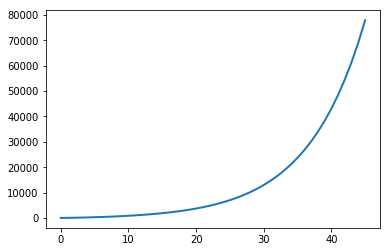❷为了计算增长率，只需要计算resize_pos数组前后两个值的商的平均值即可。

❶为了提高精度，我们只计算后半部分的平均值，注意需要用astype()方法将整数数组转换为浮点数数组。程序的输出如下：

list increase rate:

1.12754776209

【注】np.where索引定位的两种用法，np.nonzero非零值bool判断的用法，np.diff差分函数的用法。

### step3

我们可以用scipy.optimize.curve_fit()对resize_pos数组进行拟合，拟合函数为指数函数：请编写程序用上面的公式对resize_pos数组进行拟合：

```from scipy.optimize import curve_fit
#【你的程序】用指数函数对resize_pos数组进行拟合
def func(x, a, b, c, d):
return a * np.exp(b * x + c) + d
xdata = range(len(resize_pos))
ydata = resize_pos
popt, pcov = curve_fit(func, xdata, ydata)

y = [func(i, *popt) for i in xdata]
pl.plot(xdata, y, lw=1, c='r')
pl.plot(xdata, ydata, lw=1, c='b')
pl.show()
print ("list increase rate by curve_fit:")
print (10**popt)
``````list increase rate by curve_fit:
1.31158606108
```

【注意】本程序中对于scipy中的指数拟合做了示范。

### Q1：元素存储地址是否连续

首先见得的测试一下list对象存储的内容(结构3)的内存地址，

```In : a=[1,2,3,'a','b','c','de',[4,5]]

In : id(a)
Out: 139717112576840

In : for i in a:
...:     print(id(i))
...:
139717238769920
139717238769952
139717238769984
139717239834192
139717240077480
139717240523888
139717195281104
139717112078024

In : for i in a:
...:     print(id(i))
...:
139717240220952
139717240202048

In : for i in a:
...:     print(id(i))
...:
139717238770016
139717238770048
```

然后看一下相对地址，

```In : for i in a:
...:     print(id(i)-139717238769920)
...:
0
32
64
1064272
1307560
1753968
-43488816
-126691896

In : for i in a:
...:     print(id(i)-139717238769920)
...:
1451032
1432128

In : for i in a:
...:     print(id(i)-139717238769920)
...:
96
128
```

可见，对于list对象，其元素内容并不一定线性存储，但是由于内存分配的问题，会出现线性存储的假象，当元素出现容器或者相对前一个元素类型改变时，内存空间就会不再连续

### Q2：list对象地址和元素地址是否连续

其实Q1已经回答了这个问题，毕竟元素地址本身就不连续，不过我们还是测试了一下，

```In : id(a)-id(a)
Out: 126193080
```

相差甚远，而且我们分析源码可知，list对象主体是一个指针数组，也就是id(a)所指的位置主体是一个指向元素位置的指针数组，当然还有辅助的对象头信息之类的(python中几个常见的“黑盒子”之 列表list)。

### Q3：list对象（不含元素）占用内存情况分析

```In : sys.getsizeof([1,2,3,'a','b','c','de'])
Out: 120

In : sys.getsizeof([1,2,3,'a','b','c'])
Out: 112

In : sys.getsizeof([1,2,3,'a','b'])
Out: 104
```

可见，list每一个对象占用8字节32位空间，我们来看切片，

```In : sys.getsizeof(a[:3])
Out: 88

In : sys.getsizeof(a[:4])
Out: 96

In : sys.getsizeof(a[3:4])
Out: 72

In : sys.getsizeof(a[3:5])
Out: 80
```

切片对象也是每个元素占8字节，但是切片也是list对象，即使从中间切(不切头)，也会包含头信息的存储占用。

## 二、通过运算时间估算array内存分配情况

遗憾的是，无论array对象的长度是多少，sys.getsizeof()的结果都不变。因此无法用上节的方法计算array对象的增长因子。

由于内存分配时会耗费比较长的时间，因此可以通过测量每次增加元素的时间，找到内存分配时的长度。请编写测量增加元素的时间的程序：

```from array import array
import time
#【你的程序】计算往array中添加元素的时间times
times = []
times_step = []
arrays = [array('l') for i in range(1000)]
start = time.time()
for i in range(1000):
start_step = time.time()
[a.append(i) for a in arrays]
end = time.time()
times_step.append(end-start_step)
times.append(end-start)

pl.figure()
pl.plot(times)
pl.figure()
pl.plot(times_step)
pl.show()
```

输出两幅图，前面的表示元素个数对应的程序总耗时，后面的表示每一次添加元素这一过程的耗时，注意，这张图只有在array数量较大时才是这个形状，数组数量不够时折线图差异很大。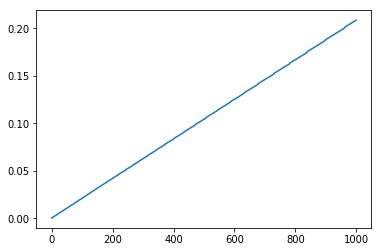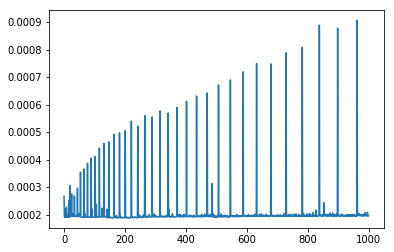进一步的，我们分析一下耗时显著大于附近点(极大值)的时刻的序列对应此时元素数量的折线图。

```ts = np.array(times_step)
le = range(np.sum(ts>0.00025))
si = np.squeeze(np.where(ts>0.00025))
pl.plot(le,si,lw=2)
pl.show()
```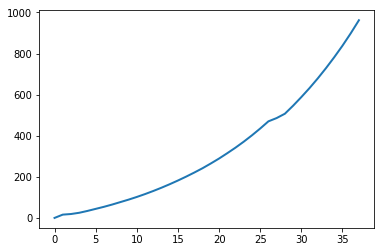### MXNet对临时数组内存的优化

以MXNet中数组为例，讲解一下序列计算时的内存变化以及优化方式，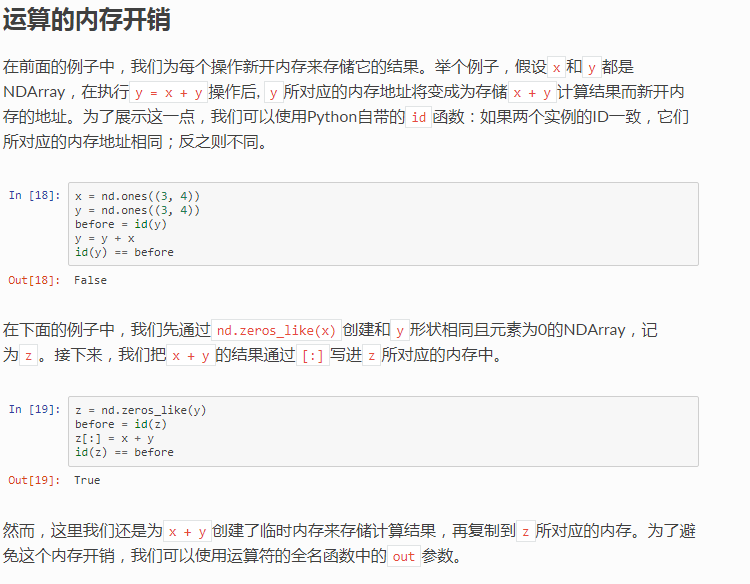• 相关阅读:
centos7 killall 命令
移动硬盘拒绝访问问题解决方法
Linux实现内容分发的主备模式的智能DNS
UWB DWM1000 跟随小车原理--- 原理代码解析
DWM1000 自动应答代码实现与实例
UWB DWM1000 跟随小车原理---一张图演示
DWM1000 帧过滤代码实现
Bphero-UWB 基站0 和 电脑串口数据格式定义
DW1000 用户手册中文版 附录3：双向测距(Two-Way Ranging)
DW1000 用户手册中文版 附录2 IEEE-802.15.4 MAC层
• 原文地址：https://www.cnblogs.com/hellcat/p/8795841.html